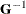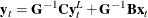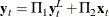# The SIMLIN Procedure

### Computing the Reduced Form

First, the SIMLIN procedure computes reduced form coefficients by premultiplying by:This can be written aswhere=C and=B are the reduced form coefficient matrices.

The reduced form matrices=C and=B are printed unless the NORED option is specified in the PROC SIMLIN statement. The structural coefficient matrices G, C, and B are printed when the ESTPRINT option is specified.